### Home > CALC > Chapter 3 > Lesson 3.2.2 > Problem3-64

3-64.

Using the definition of the derivative as a limit, show that the derivative of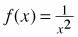is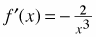. That is, show algebraically that the following is true: Homework Help ✎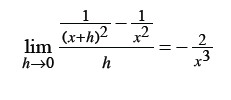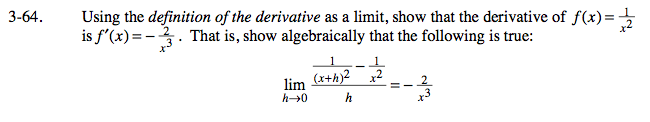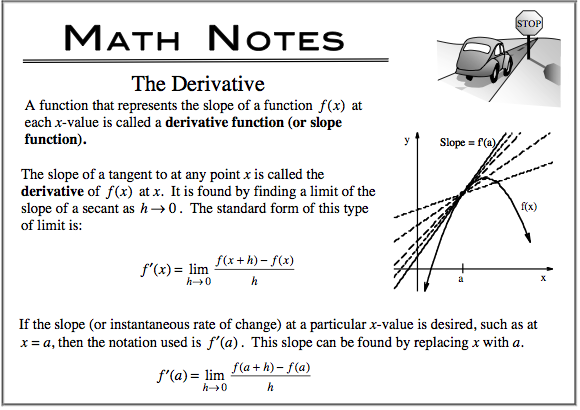This is one form of the 'definition of the derivative' (informally known as Hana's Method).

In order to evaluate this limit, we need to find an Algebraic way to cancel out the h in the denominator.

Find a common denominator in the numerator, expand and combine like terms:

Factor the numerator, then cancel out the h:

Since there is no longer and h in the denominator, you can evaluate the limit as h → 0: